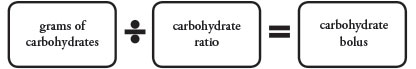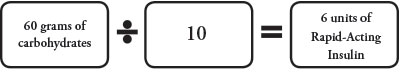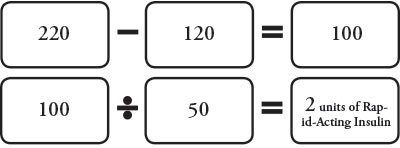# Calculating Bolus Injections

## How do I Calculate My Bolus Insulin Doses?

You will need to figure out (calculate) your bolus insulin dose based on carbohydrate eaten, blood glucose level or both added together.

### Calculating a Bolus Dose for Carbohydrate (Meal Bolus)

• Use the Insulin to Carbohydrate Ratio (ICR) to calculate your insulin dose.
• ICR is the amount of rapid-acting insulin (I) you need for a specific amount of carbohydrate (C) in food. This is the number of grams of carbohydrates that 1 unit of rapid-acting insulin will cover.
• Example: 1 unit of rapid-acting insulin will cover 10 grams carbohydrates. This may also be written 1:10.
• The ratio may be different at different meals.
• Using the ICR will control blood glucose best if insulin is given before meals. Calculate your ICR based on what you WILL be eating. We strongly suggest that rapid-acting insulin be given before meals.

NOTE: You may give insulin right after a meal if do not know the amount of carbohydrates that will be eaten, like with a very young child or sickness.

• Do not give rapid-acting insulin when you eat carbohydrate to treat a low blood glucose or to prevent a low blood glucose.### Example:

• A meal has 60 grams of carbohydrates. Your carbohydrate ratio is 1:10.
• 60 (grams of carbohydrates) divided by (÷) 10 (carbohydrate ratio) = 6 (carbohydrate bolus), so
• You would give 6 units rapid-acting insulin for carbohydrate bolus### Calculating a Bolus Dose for High Blood Glucose (Correction Bolus)

• Use the high blood glucose (hyperglycemia) correction factor, also called correction factor (CF) or sensitivity. Use this to calculate the correction bolus.
• Correction factor is how much 1 unit of rapid-acting insulin will reduce the blood glucose number.
• The target number is the blood glucose number that you want.
• If rapid-acting insulin is given with a meal, the correction dose is added to meal dose. This combined dose is rounded up or down.
• If rapid-acting insulin is given after a meal, check the blood glucose before the meal. Use that blood glucose number to calculate the correction dose.

NOTE: You should not need to correct a high blood glucose with each meal. If you need insulin to correct high blood glucose often, the insulin dose may need to be changed at each meal. The goal is to predict and prevent high blood glucose.

### Do Not Use the Correction Bolus IF:

• It has been less than 3 hours since your last meal or correction bolus.
• It has been less than 1 hour since intense exercise.
• At bedtime or during the night unless told otherwise.

### Example:

Blood glucose = 220      Target = 120         Correction Factor = 50

• 220 (actual blood glucose) - 120 (target blood glucose) = 100 (amount to correct), so
• 100 (amount to correct) ÷ 50 (correction factor) = 2 (correction bolus), so
• Give 2 units of rapid-acting insulin to bring blood glucose back into target range.### Rounding Insulin Doses

We will tell you if you need to round to whole units or half units. You may be told to use a different correction factor at bedtime or during the night.